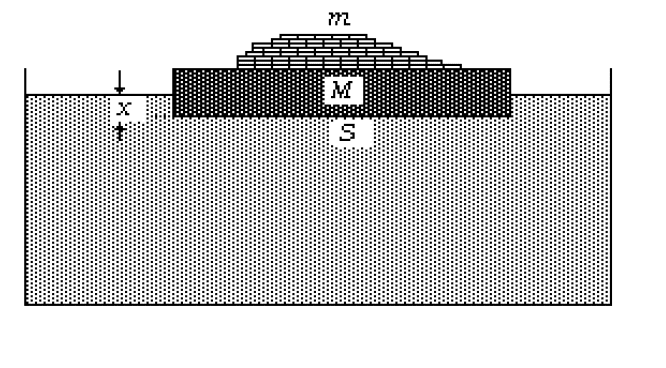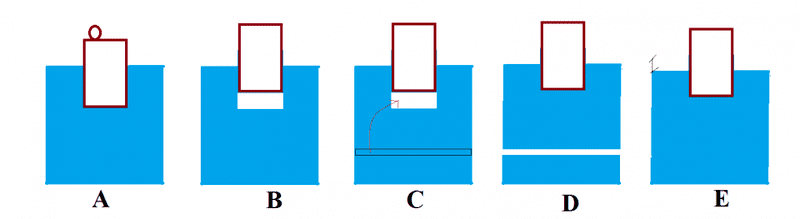# Floating block with objects that are thrown in a liquid

Soren4

## Homework Statement

I'm confused about the following kind of situation. Consider a block of density ##\rho_b##, mass ##M_b## and section ##S_b## that floats on a liquid of density ##\rho_l##, in a tank of section ##\mathcal{S}##. On the block there are some objects (all equal), of density ##\rho_o## and mass ##m_0##.Now consider two cases

1. The objects are removed from the block
2. The objects are thrown in the liquid

What does happen to the system?

Bouyancy

## The Attempt at a Solution

Case 1

Initially the block is immerged for an height ##h_1## such that $$(h_1 S_b) \rho_l= M_b + \sum m_{o}$$
When objects are removed the height of the immerged part is ##h_2## such that
$$(h_2 S_b)\rho_l= M_b$$
From here I can calculate the variation of immerged volume $$\Delta V= S_b (h_1-h_2)$$
My main question is: who does move? The liquid, the block, or both?
As far as I understood **only the liquid moves** going down, of an height ##h^*## given by
$$\Delta V=(h_1-h_2) S_b= \mathcal{h^*} \mathcal{S}$$
But I'm not convinced because maybe also the block moves, precisely it may go up. In the solution I proposed the block does not move at all, it's the liquid that moves below it. Is that correct?

Case 2

After the height ##h^*## calculated in case 1, the fluid goes up of an height ##\bar{h}## because the objects are (at least partially) immerged in the fluid.
$$\sum m_o =\rho_l \bar{h} \mathcal{S}$$
Summing up the total change i height of the fluid should be $$\Delta h= h^*- \bar{h}$$
As said above all of this assumes that only the fluid moves, while the block does not. So my question is: is that assumption correct? (And, possibly, why?)

## Answers and Replies

Hamal_Arietis
At fisrt, the objects are removed from the block, Mg<F => the block will move with acceleration F-Mg=Ma. The block moves up, then F reduces the magnitude. So the block will move. While the height of the liquid moves down too.
Actually, the block can oscillate because at equilibrium position it has inertial velocity. If you want it doesnt oscillate, implementation process (the objects are removed from the block) must be very slow.
Sorry because my English isnt good. Im very pleasure if you check my mistake

Last edited:
•Soren4
Homework Helper
Gold Member
In the solution I proposed the block does not move at all, it's the liquid that moves below it.
Consider total volume of liquid and block. Will that change?

•Soren4
Hamal_Arietis
volume of liquid dont change because we dont pour water on. But the height of liquid changes

•Soren4
Homework Helper
Gold Member
volume of liquid dont change because we dont pour water on. But the height of liquid changes
As you can see, my question was posed to Soren4. Your post #2 gave too much instruction, effectively providing the answer. The style on these forums is to nudge the student in the right direction, e.g. by asking questions that lead them to understand where their thinking is wrong. That was the aim of my question.

•Soren4
Hamal_Arietis
Sorry!The first I join this forum so I dont know this. Because Im not American so I worried that my guide makes them dont understand. So I must gave too much instruction, effectively providing the answer.

•Soren4
Homework Helper
Gold Member
Sorry!The first I join this forum so I dont know this. Because Im not American so I worried that my guide makes them dont understand. So I must gave too much instruction, effectively providing the answer.
That's ok, it's an easy mistake to make. Did you read the forum guidelines?

•Soren4
Soren4
Thanks a lot to both for your suggestions!

So actually both the block and the fluid do move.. Since my aim is to find the variation of fluid level I tried to interpret the phenomenon of case 1 as described by my answer in the question, now that I know that the block moves too. I made a drawing, maybe a little strange, I would like to ask you if that would be correct (as far as the exercise is concerned)I marked the steps with letters
• ##A##: block and objects on liquid
• ##B## : object is removed. Here i foucused only on the motion of block. The block moved up of a certain height, leaving a blank space below it (again, of course that is not true, is just to understand the exercise). The height the block moved is such that the blank volume is the one called ##\Delta V## in my question.
• ##C## : Here I imagined that I can replace ##\Delta V## with a "slice" of fluid from below (in this way I can relate ##\Delta V## to the level of the liquid in the tank of section ##\mathcal{S}##)
• ##D## : ##\Delta V## is replaced so now there is another blank volume, equal to ##\Delta V##, below
• ##E##: I imagined to move everything, as a rigid body, down, to fill the new blank volume

Total result: the height of the block and the level of the fluid changed both.

As said above this is just an attempt to justify practically my answer, after having learned that the block moves. Would you be so kind as to suggest me if this is the solution I proposed is correct even if the block moves?

Homework Helper
Gold Member
Thanks a lot to both for your suggestions!

So actually both the block and the fluid do move.. Since my aim is to find the variation of fluid level I tried to interpret the phenomenon of case 1 as described by my answer in the question, now that I know that the block moves too. I made a drawing, maybe a little strange, I would like to ask you if that would be correct (as far as the exercise is concerned)
View attachment 102591
I marked the steps with letters
• ##A##: block and objects on liquid
• ##B## : object is removed. Here i foucused only on the motion of block. The block moved up of a certain height, leaving a blank space below it (again, of course that is not true, is just to understand the exercise). The height the block moved is such that the blank volume is the one called ##\Delta V## in my question.
• ##C## : Here I imagined that I can replace ##\Delta V## with a "slice" of fluid from below (in this way I can relate ##\Delta V## to the level of the liquid in the tank of section ##\mathcal{S}##)
• ##D## : ##\Delta V## is replaced so now there is another blank volume, equal to ##\Delta V##, below
• ##E##: I imagined to move everything, as a rigid body, down, to fill the new blank volume

Total result: the height of the block and the level of the fluid changed both.

As said above this is just an attempt to justify practically my answer, after having learned that the block moves. Would you be so kind as to suggest me if this is the solution I proposed is correct even if the block moves?
Yes, that reasoning all looks correct.
How about case 2?